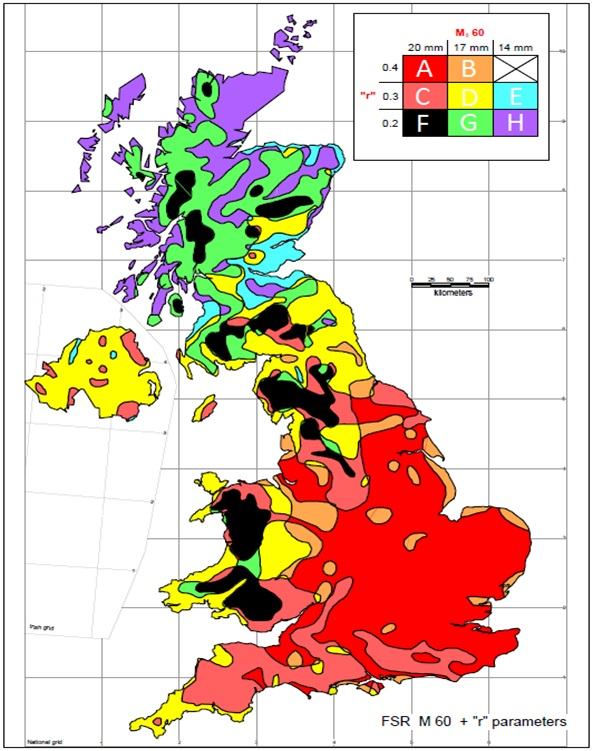# Rainfall Zone

## Rainfall Zone

The UK is divided into 8 different zones describing their rainfall characteristics. Each zone has different factors for rainfall intensity and the intensity of storm events. A map showing the different zones is given below.##### Colebrook White Pipe Design Spreadsheet

This spreadsheet uses the Colebrook-White and Darcy-Weisbach formulas to calculate the flow conditions in a circular pipe acting under gravity.

### Find Out More

This spreadsheet uses the Manning formula to calculate the flow conditions in a circular pipe acting under gravity only.

#### Manning Open Channel Design Spreadsheet

This spreadsheet uses the Manning formula to calculate the flow conditions in an open channel acting under gravity only.

This spreadsheet calculates the design runoff flow for a site in accordance with the Flood Estimation Handbook.

This spreadsheet calculates the maximum flow from a specified linear drainage channel and checks whether the channel is sufficient for the specified site and storm conditions.

This spreadsheet calculates the requirements for a attenuation system and assists the user to design a suitable system.

This spreadsheet calculates the requirements for a soakaway system and assists the user to design a suitable system.

### Full Drainage Design Suite

Full drainage design suite (50% Discount) including 7 spreadsheets;

• Colebrook White Pipe Design
• Manning Pipe Design
• Manning Open Channel Design
• Linear Drainage Design
• Runoff Calculator
• Attenuation Design
• Soakaway Design